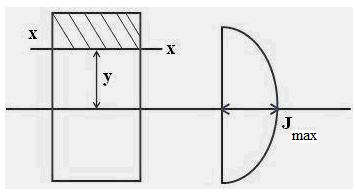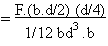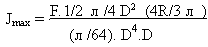#### Mechanics, Strength of Materials and Theory of Structures

SHEAR FORCE & BENDING MOMENT
PURE BENDING
SOLVED EXAMPLES BASED ON SHEAR FORCE AND BENDING MOMENT DIAGRAMS
FLEXURAL STRESS

## FLEXURAL STRESS

FLEXURAL STRESS

20.1 Introduction

Flexural strength, also known as modulus of rupture, bend strength, or fracture strength a mechanical parameter for brittle material, is defined as a material's ability to resist deformation under load. The transverse bending test is most frequently employed, in which a rod specimen having either a circular or rectangular cross-section is bent until fracture using a three point flexural test technique. The flexural strength represents the highest stress experienced within the material at its moment of rupture. It is measured in terms of stress, here given the symbol σ.Fig. 20.1 Beam diagram

20.2 Flexural Stress

When a member is being loaded similar to that in figure 20.1 flexure stress (or bending stress) will result. Bending stress is a more specific type of normal stress. When a beam experiences load like that shown in figure 20.1 on the top fibers of the beam undergo a normal compressive stress. The stress at the horizontal plane of the neutral axis is zero. The bottom fibers of the beam undergo a normal tensile stress. It can be concluded therefore that the value of the bending stress will vary linearly with distance from the neutral axis.

Calculating the maximum bending stress is crucial for determining the adequacy of beams, rafters, joists, etc.

σb =MY/I

σb=Bending stress

M=Calculated Bending Moment, M.m

Y=Vertical distance away from neutral axis,

I=Moment of inertia about the neutral axis, m4

20.3 Shear Stresses in Beams Relations between Centre, Torsional and Flexural Loads

The shear stress in any section at a distance ’y’ from neutral axis is given by

σavg = F / b d

Jmax = FAy / IbFig. 20.2 Shear stress distribution in rectangular section

Where

σavg = Complimentary (or) Horizontal shear

stress at a layer x – x.

F = Shear Force at a section, M

A = Area above y below the section x – x, m2

y = Distance of centroid of area ‘A’ from neutral axis ,m

I = Moment of Inertia about the neutral axis, m4

b = Width of section x – x , m

Average shear stress is given by

σAvg = F / A

Where F = Shear force

A = Cross sectional Area

For Rectangular cross sectionFig. 20.3 Shear stress distribution in rectangular section

σavg = F/ b.d σmax = FAy /I * b

l =1/12 b.d3= 3/2 F/b.d

σmax = 3/2 σavg

For square cross section

Same as rectangular cross section.

σmax = 3/2 σavgFig. 20.4 Shear stress distribution in square section

For Circular cross section

Y = 4R/3л

σavg = F/ (л /4)D2σmax= 4/3 σavg.Fig. 20.5 Shear stress distribution in circular section

Note:

A cross section having more area at N.A is strong in resisting shear. {except circle}.

If bending and shear are acting together in a beam, the main design criterion is the bending only. Therefore a rectangular section is commonly used as a beam.